Tourist Jirka

Distance between the points A and B is 13.5 km. Jirka went from point A to point B unknown speed and for an unknown period of time. Back to the point A went slower by 3 km/h which means that went 20 minutes more.

How long Jirka took the return journey?

Result

t2 =  1.4 h

Solution:Leave us a comment of this math problem and its solution (i.e. if it is still somewhat unclear...):Be the first to comment!To solve this verbal math problem are needed these knowledge from mathematics:

Looking for help with calculating roots of a quadratic equation? Do you have a linear equation or system of equations and looking for its solution? Or do you have quadratic equation? Do you want to convert length units?

Next similar math problems:

1. Two citiesCities A and B are 200 km away. At 7 o'clock from city A, the car started at an average speed of 80 km/h, and from B at 45 min later the motorcycle is started at an average speed of 120 km/h. How long will they meet and at what distance from the point A it
2. Sebastian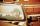From Kovalov Sebastian walks at 6 km / h started at 8:00 in the direction of Kuty. From Kuty, the godfather is driving at 50 km/h and started at 8:30. The distance is 24 km. When and where grandfather will take Sebastian to the car.
3. Warehouse carsFrom the warehouse started truck at speed 40km/h. After 1hour 30mins started from the same place same direction a car at speed 70 km/h. For how long and at what distance from the warehouse overtake a truck?
4. Cycling trip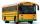At 8 am started a group of children from the camp to day cycling trip. After 9:00 weather deteriorated sharply and the leader decided to send for the children bus on the same route, which started at 10 hours. For how long and at what distance from the camp
5. Wind drift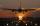The plane flies at 860 km/h, passing distance 3000 kilometers with the wind and once again against the wind for 6 h 59 min. What is the wind speed?
6. Moving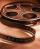Vojta left the house at three o'clockat 4 km/h. After half hour later went from the same place Filip by bicycle at speed 18 km/h. How long take Tilip to catch up Vojta and how far from the house?
7. Theorem proveWe want to prove the sentence: If the natural number n is divisible by six, then n is divisible by three. From what assumption we started?
8. TrainsFrom station 130 km away started passenger train and after 2.2 hours after the express train, which travels 37 km an hour more. Express train finish journey 7 minutes early. Calculate the average speed of this two trains.
9. Steamer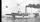At 6 hours 40 minutes steamer sailed from the port at speed 12 km/h. At exactly 10 hours started sail motorboat at speed 42 km/h. When motorboat will catch steamer?
10. The cruise ship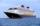The cruise ship has a speed of 12 km / h at a calm surface. When we sail 45 km along the river and 45 km back, it took us exactly 8 hours. Which (constant) speed of flow of the river?
11. DiscriminantDetermine the discriminant of the equation: ?
12. Solve 3Solve quadratic equation: (6n+1) (4n-1) = 3n2Find the roots of the quadratic equation: 3x2-4x + (-4) = 0.Equation ? has one root x1 = 8. Determine the coefficient b and the second root x2.Determine the quadratic equation absolute coefficient q, that the equation has a real double root and the root x calculate: ?Calculate the wavelength of the tone frequency 11 kHz if the sound travels at speeds of 343 m/s.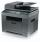The new copier copying a folder of papers 5 min. faster than the old. The operator used new, but out of toner and exchange took 5 min. In that time copied on the old. The whole work has been done for 9 min. How long would the work done only by old copier?